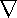# Maths - Curl

Curl takes a vector field as an input and gives another vector field as an output. Curl is a measure of rotation where the which points away from the vortex according to the right-hand rule. So divergence tells us how much is going in or out of our infinitesimally small volume, curl tells how much things are going around the volume.

If the vector field represents velocity, then curl of this will be 2 times the angular velocity. This page shows this for a rotating solid object.

We have to be a bit careful about what we mean by rotation here, it is not just circular movement that will produce a non-zero curl value, imagine we have a fluid flowing through a pipe in such a way that it is flowing faster in the centre of the pipe than outside, this will also produce a non-zero curl value. To visualise this imagine an infinitesimally small paddle wheel in the fluid, if this would be rotated then curl is non-zero.

Another way to look at it is, curl tells you how much the rate of change of a function in one direction changes as you move in a different direction.

Curl is defined, in the 3D case (there are relatives to curl for more or less dimensions), as follows:

curl()=×=
 ∂vz - ∂vy ∂y ∂z
 ∂vx - ∂vz ∂z ∂x
 ∂vy - ∂vx ∂x ∂y

Where:

• vx = a scalar representing the component of v in the x direction.
• vy = a scalar representing the component of v in the y direction.
• vz = a scalar representing the component of v in the z direction.×is an alternative way to denote curl(v), where:

•= is the vector operator as described here
• × = is the cross product as described here
• v = is the vector being operated on

Or in alternative notation:

 curl(v) = i( ∂vz - ∂vy )+ k( ∂vx - ∂vz )+ j ( ∂vy - ∂vx ) ∂y ∂z ∂z ∂x ∂x ∂y

Another way to write this is as a determinant as follows:

curl(v)=×v =
i j k
 ∂ ∂x
 ∂ ∂y
 ∂ ∂z
∂x vy vz

# Identities

curl(s v) = s curl(v) + (curl(s)) × v

curl(v1× v2) = (v2) v1 - (v1) v2 + v1 div v2 + v2 div v1